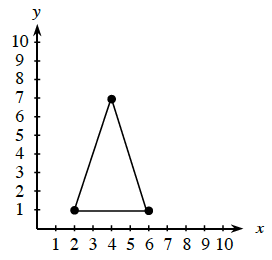### Home > CC1MN > Chapter 1 Unit 3B > Lesson CC2: 1.2.7 > Problem1-127

1-127.

Locate the coordinates of the three highlighted points on the graph of the triangle at right and write them as ordered pairs $(x,y)$.The $x$ coordinate is the number of places going right or left to the point.
The $y$ coordinate is the number of places going up or down to the point.

One ordered pair is $(2,1)$.

Don't forget to put the $x$ coordinate before the $y$ coordinate in your ordered pair. $(x,y)$